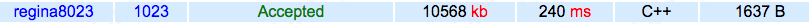# 【BZOJ 1023】 [SHOI2008]cactus仙人掌图

## 1023: [SHOI2008]cactus仙人掌图

Time Limit: 1 Sec   Memory Limit: 162 MB
Submit: 1235   Solved: 482
[ Submit][ Status]

## Description## Sample Input

15 3
9 1 2 3 4 5 6 7 8 3
7 2 9 10 11 12 13 10
5 2 14 9 15 10 8
10 1
10 1 2 3 4 5 6 7 8 9 10

9

## HINT【注意】使用Pascal语言的选手请注意：你的程序在处理大数据的时候可能会出现栈溢出。如果需要调整栈空间的大小，可以在程序的开头填加一句：{\$M 5000000}，其中5000000即指代栈空间的大小，请根据自己的程序选择适当的数值。

f[x]表示以x为根的子树的最长链长度

（1）dfn[x]<low[y]。

（2）dfn[x]>=low[y]

fa[y]!=x,dfn[x]<dfn[y]。

#include <iostream>
#include <cstring>
#include <algorithm>
#include <cstdio>
#include <cstdlib>
#define M 200005
using namespace std;
int num=0,ans=0,n,m,tot,h[M],f[M],low[M],dfn[M],q[M],a[M],fa[M];
struct edge
{
int y,ne;
}e[M];
{
tot++;
e[tot].y=y;
e[tot].ne=h[x];
h[x]=tot;
}
void dp(int x,int y)
{
int m=0;
while (y!=x)
{
a[++m]=f[y];
y=fa[y];
}
a[++m]=f[x];
for (int i=1;i<m;i++)
a[m+i]=a[i];
int l,r;
int p=m/2;
q[l=r=1]=1;
for (int i=2;i<=m+p;i++)
{
while (l<=r&&i-q[l]>p)
l++;
ans=max(ans,a[q[l]]+a[i]+i-q[l]);
while (l<=r&&a[q[r]]+i-q[r]<=a[i])
r--;
q[++r]=i;
}
for (int i=1;i<m;i++)
f[x]=max(f[x],a[i]+min(i,m-i));
}
void tarjan(int x)
{
dfn[x]=low[x]=++num;
for (int i=h[x];i;i=e[i].ne)
if (fa[x]!=e[i].y)
{
if (!dfn[e[i].y])
{
int y=e[i].y;
fa[y]=x;
tarjan(y);
low[x]=min(low[x],low[y]);
if (dfn[x]<low[y])
{
ans=max(ans,f[x]+f[y]+1);
f[x]=max(f[x],f[y]+1);
}
}
else low[x]=min(low[x],dfn[e[i].y]);
}
for (int i=h[x];i;i=e[i].ne)
if (fa[e[i].y]!=x&&dfn[x]<dfn[e[i].y])
dp(x,e[i].y);
}
int main()
{
scanf("%d%d",&n,&m);
for (int i=1;i<=m;i++)
{
int k,x,y;
scanf("%d%d",&k,&x);
for (int j=1;j<k;j++)
{
scanf("%d",&y);
x=y;
}
}
tarjan(1);
cout<<ans<<endl;
return 0;
}1.对tarjan求连通分量有了更深入的理解~点双连通分量相当于找环，通过儿子的low来判断当前点是否是割点。

2.lyd博客

11-03
01-101607
08-19
02-05
08-21
11-04
10-13
11-04
11-04
01-30
09-14
12-04
11-26
12-20
08-01

### “相关推荐”对你有帮助么？

•非常没帮助
•没帮助
•一般
•有帮助
•非常有帮助被折叠的  条评论 为什么被折叠?到【灌水乐园】发言Regina8023

¥2 ¥4 ¥6 ¥10 ¥20余额支付 (余额：-- )扫码支付获取中扫码支付点击重新获取扫码支付1.余额是钱包充值的虚拟货币，按照1:1的比例进行支付金额的抵扣。
2.余额无法直接购买下载，可以购买VIP、C币套餐、付费专栏及课程。余额充值